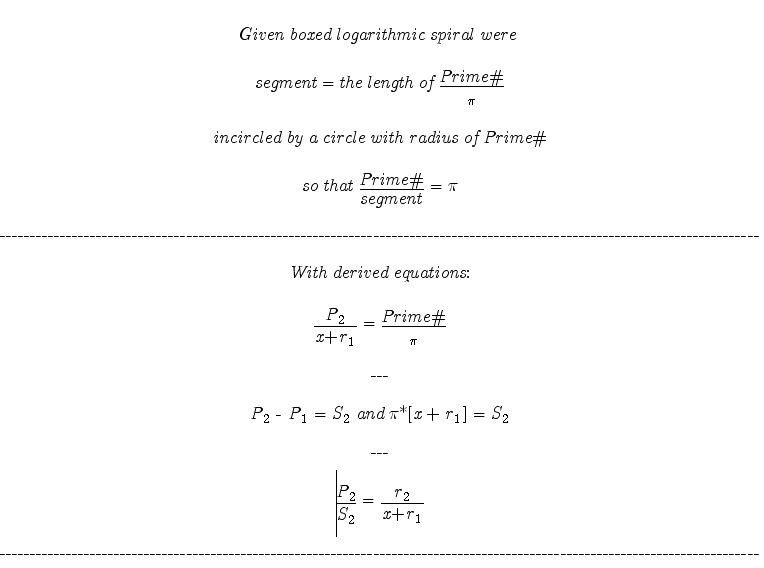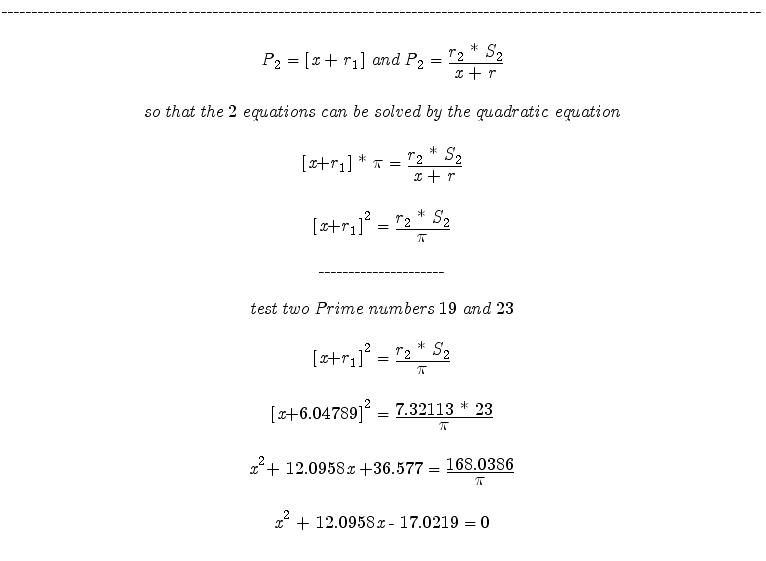_______ 20070609 Here there are 2 logarithmic spirals that are based on Prime numbers. The one the first set of equations are based on Click Here and the second: ... drawn in boxed construction... with the segment = the radius of (Prime# / Pi) (Note this logarithmic spiral was originally posted in May2007.) So there are 2 known log spirals which we know much information about. We can use this knowledge to combine equations and solve for Prime numbers. Note this solution will only use the basics of Algebra and Trigonometry. I tested well over 500 equations. Only the correct ones will be shown here. Proof of the theories and work will be added over time.I will be adding more content to support this work soon. However do not wait and test the theory for yourself. May the Creative Force be with You!